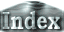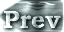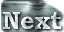Horizontal scales of convection cells (1)From the numerical calculations and theoretical considerations, it has been shown that the average temperature of the fluid is higher when a plate covers whole the surface of the domain. If such an effect also occurs when a plate covers only part of the surface, then a horizontal temperature difference between points underneath and outside the plate is generated. This temperature difference is expected to induce another kind of a fluid flow. When the Rayleigh number is increased, the temperature difference also increases according to Figure 8, and the newly established fluid flow regime is expected to intensify. We expect that the difference in the horizontal scales of convection cells in the case of Ra=104 and the cases of Ra=105 and 106 revealed by the numerical calculations is due to the differences in the horizontal temperature effect. In the following, we discuss the horizontal scale of convection cells by estimating this effect.

 Figure 9: An illustration of the temporal development of convection.In order to estimate the horizontal scale of convection cells, we consider a convection development scenario incorporating the following features:

• Initially, convection develops in response to the vertical temperature difference imposed at the boundaries.
• Due to the presence of the plate, a temperature difference between points beneath and outside the plate is established by the convection. This horizontal temperature difference causes another mode of a fluid flow.
• If the velocity amplitude of the newly established circulation uh is larger than that of the intrinsic thermal convection u and u', then convection cells of large horizontal sizes will appear.
• On the other hand, if uh is smaller than u and u', then convection cells of large horizontal sizes will not develop.

If we accept this scenario, then we can deduce that the convection cells of large horizontal scales observed for Ra=105 and 106 correspond to those induced by the horizontal temperature difference associated with the plate (uh > u,u'), while the convection cells of aspect ratio 1.5 in the case of Ra=104 correspond to the situation in which the effect of the horizontal temperature difference is small (uh < u,u') .

 Horizontal scales of convection cells (1)